Vectors and conics

This free course is available to start right now. Review the full course description and key learning outcomes and create an account and enrol if you want a free statement of participation.

Free course

1.8 Intersection of two planes

We saw earlier that two arbitrary lines in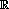2 may intersect, be parallel, or coincide. In an analogous way, two arbitrary planes in3 may intersect, be parallel, or coincide.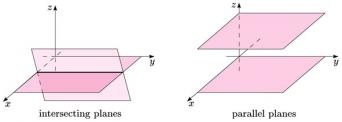In general, if two distinct planes intersect, then the set of common points is a line that lies in both planes.

For example, the (x, y)-plane and the (x, z)-plane intersect in the x-axis, which lies in both planes.

Similarly, the planes xy = 0 and x + y + z = 1 intersect in a line; the points (x, y, z) on this line all satisfy both of the equations

xy = 0   and   x + y + z = 1.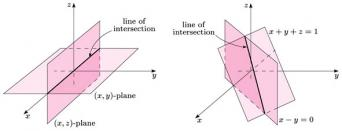Two planes in3 may be parallel, and so cannot intersect. For example, the plane with equation z = 0 is the (x, y)-plane, and the plane with equation z = 1 is a plane parallel to the (x, y)-plane, passing through the point (0, 0, 1) on the z-axis. These two planes do not intersect; every point in the plane z = 1 lies at distance 1 above the plane z = 0.

Finally, two planes may coincide. For example, the planes with equations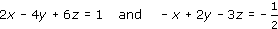coincide, since the second equation is simply the first multiplied by the number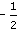.

In general, two planes are coincident if the equation of one can be rearranged to be a multiple of the equation of the other.

Example 10

Determine whether the planes with equations z = 2 and y = −1 intersect, are parallel, or coincide. Illustrate your answer with a sketch.

Hint: You have already sketched these two planes in Exercise 8.

From the sketches in Exercise 8, the two planes are clearly not parallel or coincident; hence they must intersect in a line that lies in both planes.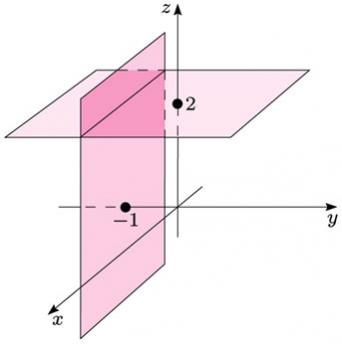M208_1